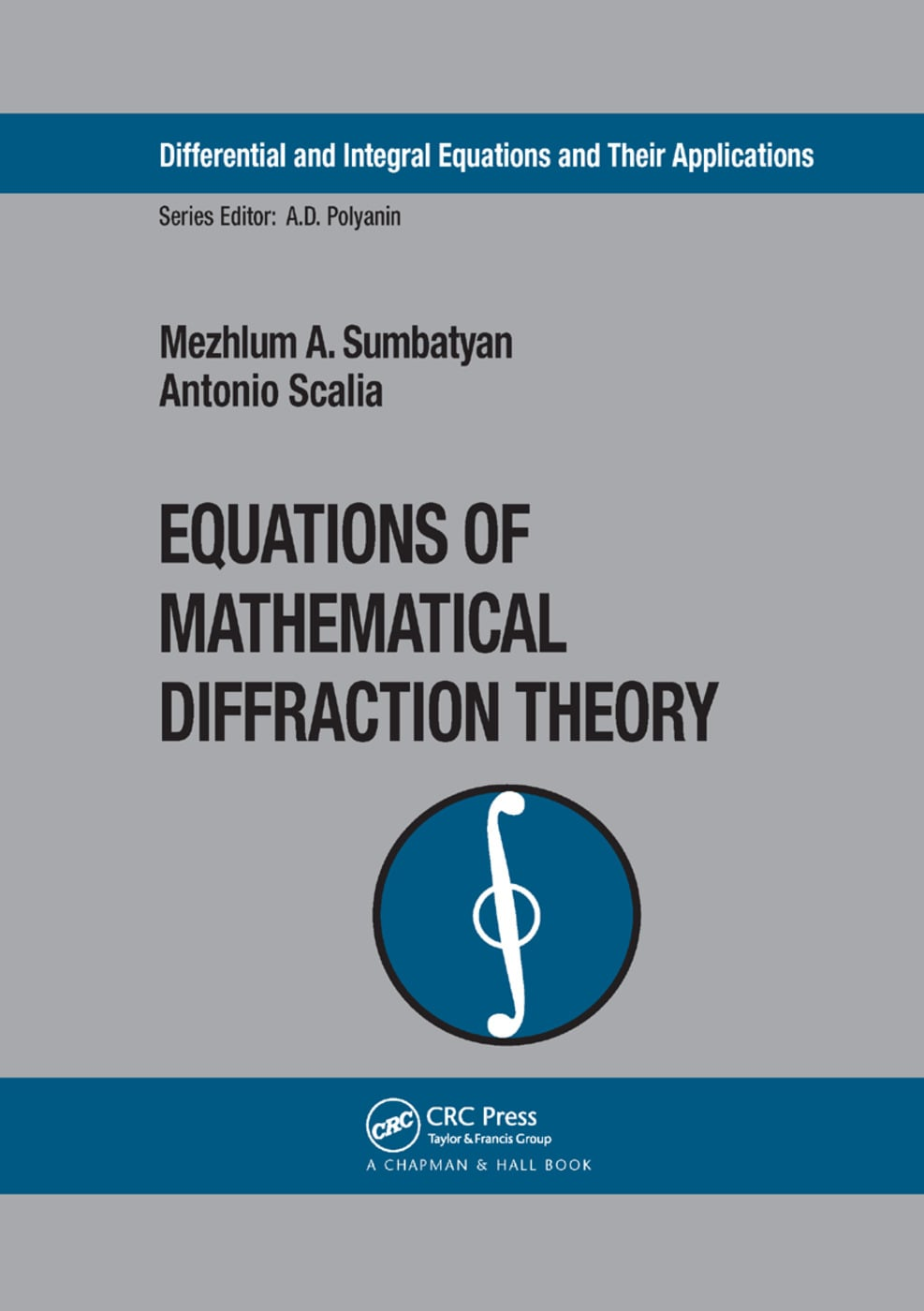# Equations of Mathematical Diffraction Theory

## 1st Edition

CRC Press

312 pages

Paperback: 9780367393809
pub: 2019-06-19
\$74.95
x
Hardback: 9780415308496
pub: 2004-09-29
Currently out of stock
\$175.00
x

FREE Standard Shipping!

### Description

Equations of Mathematical Diffraction Theory focuses on the comparative analysis and development of efficient analytical methods for solving equations of mathematical diffraction theory. Following an overview of some general properties of integral and differential operators in the context of the linear theory of diffraction processes, the authors provide estimates of the operator norms for various ranges of the wave number variation, and then examine the spectral properties of these operators. They also present a new analytical method for constructing asymptotic solutions of boundary integral equations in mathematical diffraction theory for the high-frequency case.

Clearly demonstrating the close connection between heuristic and rigorous methods in mathematical diffraction theory, this valuable book provides you with the differential and integral equations that can easily be used in practical applications.

Some Preliminaries from Analysis and the Theory of Wave Processes. Integral Equations of Diffraction Theory for Obstacles in Unbounded Medium. Wave Fields in a Layer of Constant Thickness. Analytical Methods for Simply Connected Bounded Domains. Integral Equations in Diffraction by Linear Obstacles. Short-Wave Asymptotic Methods on the Basis of Multiple Integrals. Inverse Problems of the Short-Wave Diffraction. Ill-Posed Equations of Inverse Diffraction Problems for Arbitrary Boundary. Numerical Methods for Irregular Operator Equations.

Sumbatyan, Mezhlum A.; Scalia, Antonio